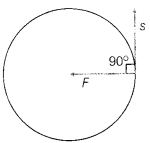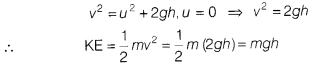# By an electron revolving in a circular orbit of radius V around a nucleus

What is the amount of work done in the following cases? Justify your answer by giving the appropriate reason.

1. By an electron revolving in a circular orbit of radius V around a nucleus.
2. By the force of gravity, when a stone of mass ‘m is dropped from the top of a multistoreyed building of height ‘h’.
1. When an electron revolves around the nucleus in circular orbit, a centripetal force is acting inwards while it displaces tangentially at angle of 90° on its circumference as shown, so work done is zero. i.e. W = Fs cos 90°2. As the stone is dropped from rest, its potential energy starts converting into KE. Let its speed be v, then from